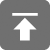# 工学1号馆

home

## Project Euler 21–Amicable numbers

Wu Yudong    August 12, 2018     欧拉计划   446

Let d(n) be defined as the sum of proper divisors of n (numbers less than n which divide evenly into n). If d(a) = b and d(b) = a, where ab, then a and b are an amicable pair and each of a and b are called amicable numbers.

For example, the proper divisors of 220 are 1, 2, 4, 5, 10, 11, 20, 22, 44, 55 and 110; therefore d(220) = 284. The proper divisors of 284 are 1, 2, 4, 71 and 142; so d(284) = 220.

Evaluate the sum of all the amicable numbers under 10000.

d(n)定义为n 的所有真因子（小于 n 且能整除 n 的整数）之和。 如果 d(a) = b 并且 d(b) = a, 且 ab, 那么 a 和 b 就是一对相亲数（amicable pair），并且 a 和 b 都叫做亲和数（amicable number）。

#include<stdio.h>

int FactorSum(int n)			//计算n的所有小于n的因素和
{
int i;
int sum = 1;
for (i = 2; i <= n / 2; i++) {
if (n % i == 0)
sum += i;
}
return sum;
}

int main()
{
int t, i = 2;
int sum = 0;
while (i < 10000) {
t = FactorSum(i);
if (t != i && FactorSum(t) == i)
sum += i;
i++;
}
printf("%d\n", sum);
return 0;
}